## 0x01 JD-GUI的用途与缺陷：

JD-GUI是一款从JAVA字节码中还原JAVA源代码的免费工具，一般情况下使用这款工具做JAVA逆向就足够了，但是由于其原理是从JAVA字节码中按照特定结构来还原对应的JAVA源代码，因此一旦字节码结构被打乱（比如说使用混淆器），那么JD-GUI就会失去它的作用，如图为使用JD-GUI打开Burpsuite时的显示：## 0x02 字节码分析：

Java的字节码并不像普通的二进制代码在计算机中直接执行，它通过JVM引擎在不同的平台和计算机中运行。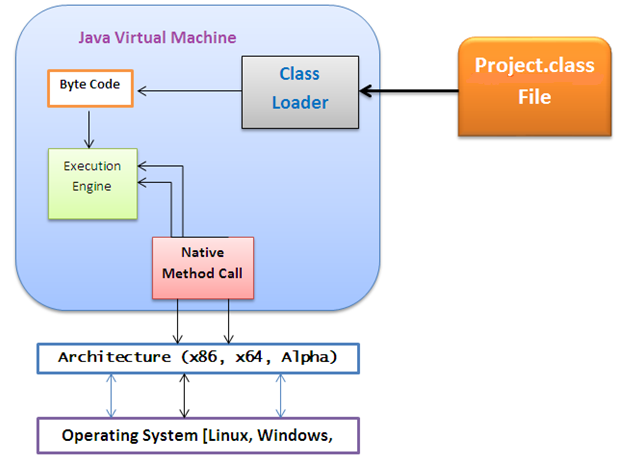JVM是一个基于栈结构的虚拟计算机，使用的是JVM操作码（及其助记符），在这一点上和普通二进制反汇编的过程非常相似。 对Java字节码进行反编译其实非常简单，JDK内置的Javap工具即可完成这项任务。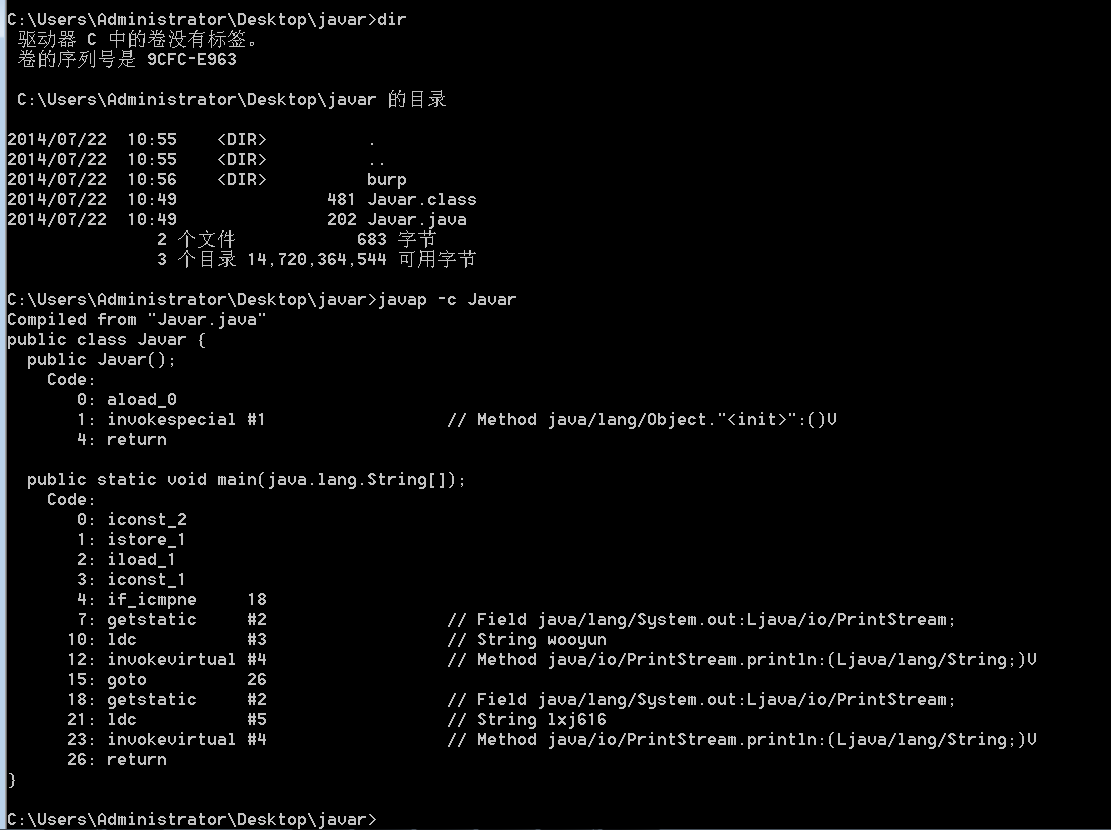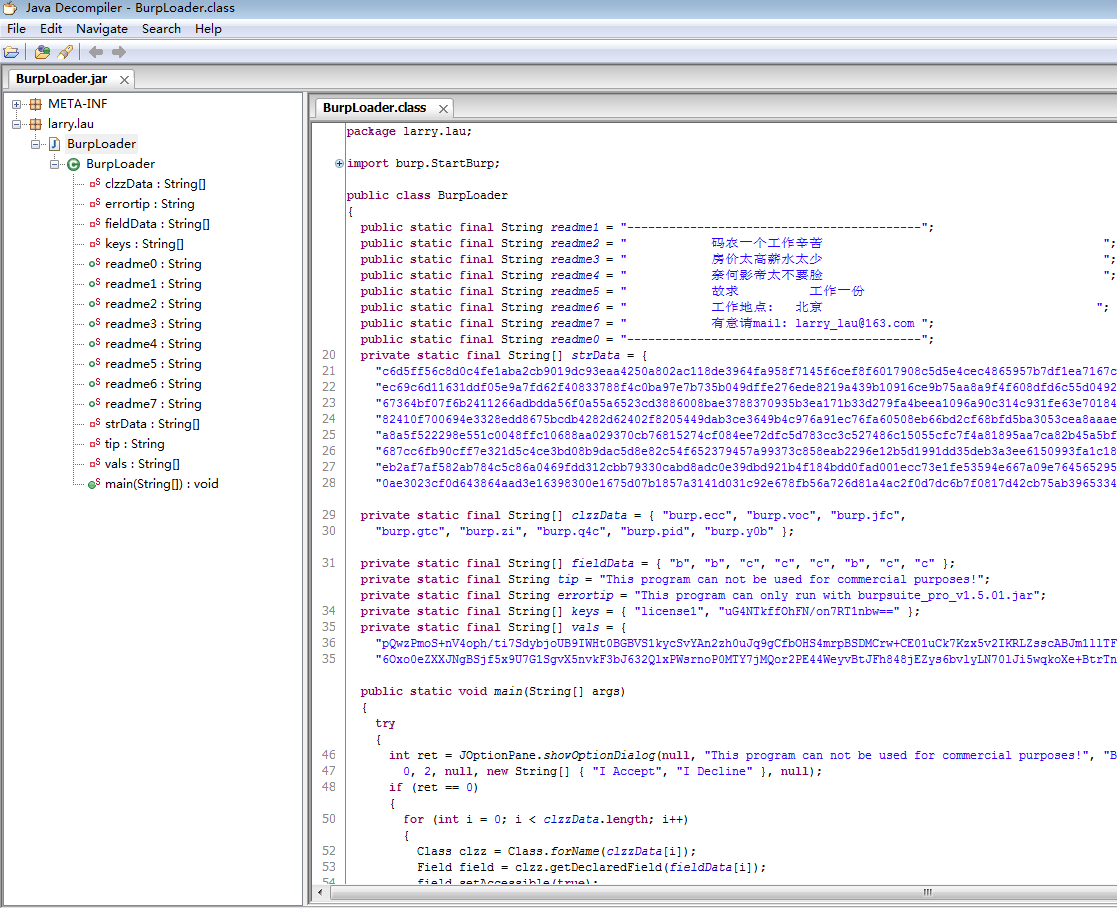``````public static void main(String[] args)
{
try
{
int ret = JOptionPane.showOptionDialog(null, "This program can not be used for commercial purposes!", "BurpLoader by [email protected]/* <![CDATA[ */!function(t,e,r,n,c,a,p){try{t=document.currentScript||function(){for(t=document.getElementsByTagName('script'),e=t.length;e--;)if(t[e].getAttribute('data-cfhash'))return t[e]}();if(t&#038;&#038;(c=t.previousSibling)){p=t.parentNode;if(a=c.getAttribute('data-cfemail')){for(e='',r='0x'+a.substr(0,2)|0,n=2;a.length-n;n+=2)e+='%'+('0'+('0x'+a.substr(n,2)^r).toString(16)).slice(-2);p.replaceChild(document.createTextNode(decodeURIComponent(e)),c)}p.removeChild(t)}}catch(u){}}()/* ]]&gt; */", 0, 2, null, new String[] { "I Accept", "I Decline" }, null);
//显示选择对话框：这程序是出于学习目的写的，作者邮箱larry_lau(at)163.com
if (ret == 0)  //选择我同意
{
//以下用到的是java反射机制，不懂反射请百度
for (int i = 0; i < clzzData.length; i++)
{
Class clzz = Class.forName(clzzData[i]);
//是burpsuite的静态类（名字被混淆过了，也没必要列出了）
Field field = clzz.getDeclaredField(fieldData[i]);
//静态类中的变量也被混淆过了，也不必列出了
field.setAccessible(true);
//访问private必须先设置这个，不然会报错

field.set(null, strData[i]);
//把变量设置成strData（具体那一长串到底是什么暂不讨论）
}

Preferences prefs = Preferences.userNodeForPackage(StartBurp.class);
//明显preferences是用来存储设置信息的
for (int i = 0; i < keys.length; i++)
{
// key和val能猜出是什么吧
String v = prefs.get(keys[i], null);
if (!vals[i].equals(v))
{
prefs.put(keys[i], vals[i]);
}
}
StartBurp.main(args);
}
}
catch (Exception e)
{
JOptionPane.showMessageDialog(null, "This program can only run with burpsuite_pro_v1.5.01.jar", "BurpLoader by [email protected]/* <![CDATA[ */!function(t,e,r,n,c,a,p){try{t=document.currentScript||function(){for(t=document.getElementsByTagName('script'),e=t.length;e--;)if(t[e].getAttribute('data-cfhash'))return t[e]}();if(t&#038;&#038;(c=t.previousSibling)){p=t.parentNode;if(a=c.getAttribute('data-cfemail')){for(e='',r='0x'+a.substr(0,2)|0,n=2;a.length-n;n+=2)e+='%'+('0'+('0x'+a.substr(n,2)^r).toString(16)).slice(-2);p.replaceChild(document.createTextNode(decodeURIComponent(e)),c)}p.removeChild(t)}}catch(u){}}()/* ]]&gt; */",
0);
}
}
}
``````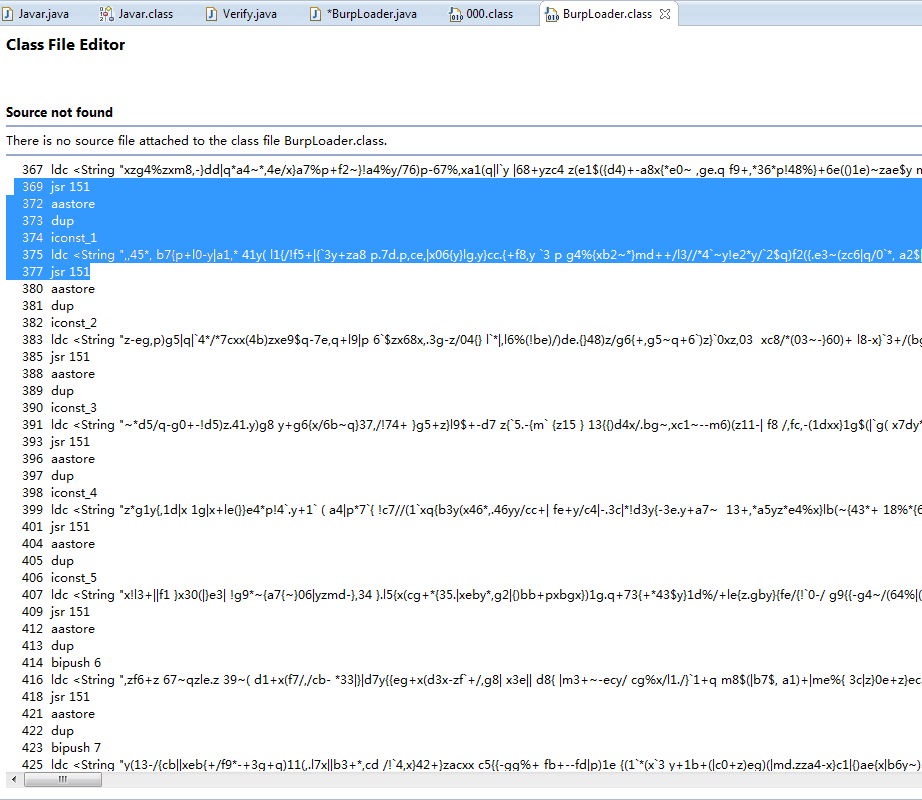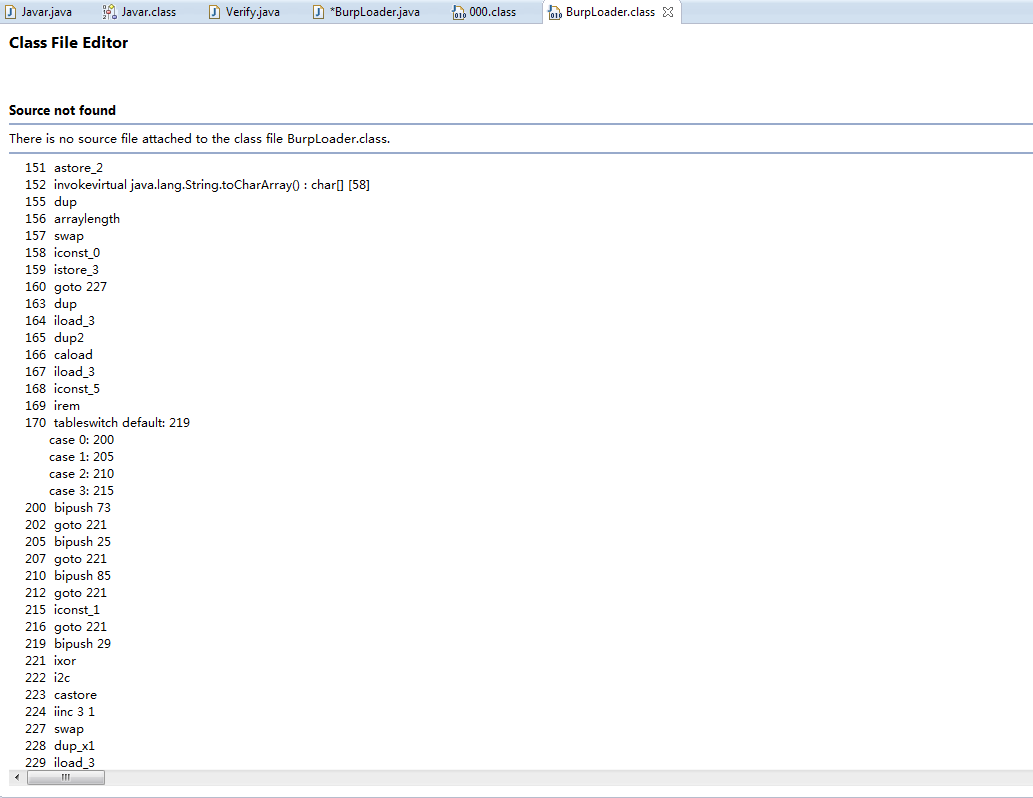``````public class Verify {
private static String decrypt(String str) {
char key[] = new char[] {73,25,85,1,29};
char arr[] = str.toCharArray();
for (int i = 0; i < arr.length; i++) {
arr[i] ^= key[i % 5];
}
return new String(arr);
}

public static void main (String args[]) {
System.out.println(decrypt("%x'sdgu4t3#x#`egj\"hs.7%m|/7;hp+l&/S t7tn\5v:j\'}_dx%"));
}
}
``````

## 0x05 总结&POC

``````package stratburp;

import burp.StartBurp;
import java.lang.reflect.Field;
import java.util.prefs.Preferences;
import javax.swing.JOptionPane;

public class startburp
{

private static final String[] clzzData = { "burp.ecc", "burp.voc", "burp.jfc",
"burp.gtc", "burp.zi", "burp.q4c", "burp.pid", "burp.y0b" };

private static final String[] fieldData = { "b", "b", "c", "c", "c", "b", "c", "c" };
private static final String errortip = "This program can only run with burpsuite_pro_v1.5.01.jar";
private static final String[] keys = { "license1", "uG4NTkffOhFN/on7RT1nbw==" };

public static void main(String[] args)
{
try
{
for (int i = 0; i < clzzData.length; i++)
{
Class clzz = Class.forName(clzzData[i]);
Field field = clzz.getDeclaredField(fieldData[i]);
field.setAccessible(true);

//field.set(null, strData[i]);
System.out.println(field.get(null));
}

Preferences prefs = Preferences.userNodeForPackage(StartBurp.class);
for (int i = 0; i < keys.length; i++)
{
String v = prefs.get(keys[i], null);
System.out.println(prefs.get(keys[i], null));
}
StartBurp.main(args);
}
catch (Exception e)
{
JOptionPane.showMessageDialog(null, "This program can only run with burpsuite_pro_v1.5.01.jar", "Notice",0);
}
}
}
``````